# Fitting FITS Image DataSherpa Threads (CIAO 4.11 Sherpa v1)

## Overview

#### Synopsis:

This thread models image data of the supernova remnant G21.5-0.9 using a source model expression that involves multiple model components. The Create and Read Fits Image Data section shows how the input file was created.

Last Update: 12 Dec 2018 - reviewed for CIAO 4.11, no content change

## Create and Read Fits Image Data

`unix% download_chandra_obsid 1838 `

In this example, the data has been reprocessed with chandra_repro. First, an image of the region of interest is created:

```unix% punlearn dmcopy
unix% dmcopy \
"acisf01838_repro_evt2.fits[sky=box(4059.25,4235.75,521.5,431.5)][bin x=::2,y=::2]" \
image2.fits
```

Because we plan to model the extended structure, not detailed features in the image, we can use coarser binning to reduce the time taken for the fit—a binning factor of 2 is used. The choice of binning should be determined by the quality of the data and the models to be fit with the data. Increasing the binning factor when creating the image is likely to lose small-scale spatial information.

After starting a Sherpa session, the dataset is input using the load_image command. The image is then displayed by the image_data command:

```sherpa> load_image("image2.fits")
sherpa> image_data()
```

which creates Figure 1. The DS9 imager is used by default; see the Using SAOImage DS9 CIAO thread for more information.

### Figure 1: Image of the data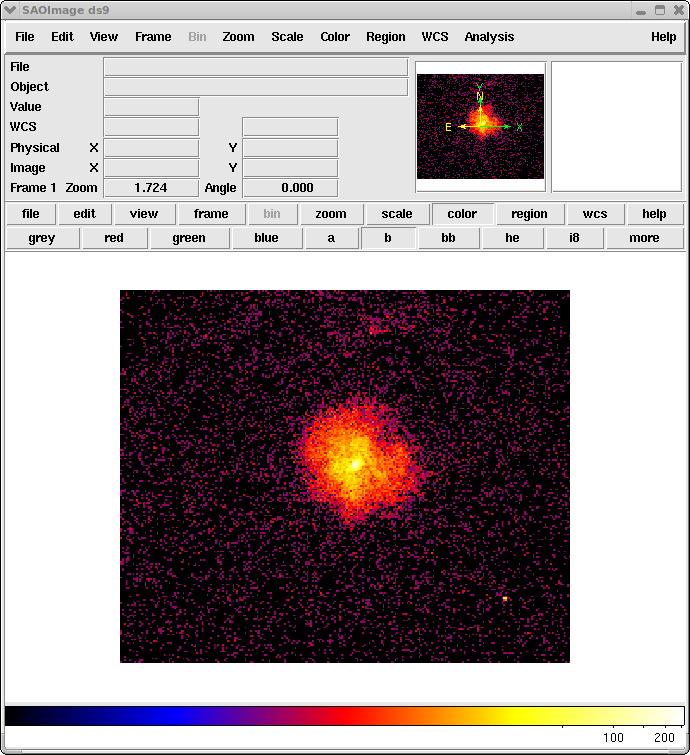### Figure 1: Image of the data

Note that a logarithmic scaling has been chosen to bring out the faint emission of the source.

### Setting the coordinate system

The default coordinate system for fitting images is that of logical (also called image), which uses the FITS convention for numbering pixels: the first pixel is centered at (1,1) with the bottom-left corner being at (0.5,0.5) and the top-right corner being at (1.5,1.5).

Although a simple system, it can be difficult when converting the best-fit parameters, such as source location, back to more-meaningful coordinate systems. For this reason, the physical coordinate system is chosen for fitting. This is done with the set_coord function:

```sherpa> set_coord("physical")
```

### Choosing the appropriate statistics

When fitting Chandra or XMM-Newton imaging data, it is almost certain that many pixels will only contain a small number of counts, making the source model fit with Cash statistic, the Poisson log-likelihood, to the Poisson image suitable. Subsequently, subtracting a background data set is not permitted and must either be accounted for with a background model—as shown in this example—or the background signal is ignored.

```sherpa> set_stat("cash")
```

The CSTAT statistic—equivalent to the XSPEC C statistic—may also be used in fitting image data. The advantage of CSTAT over CASH is that an approximate goodness-of-fit measure may be assigned to a given value of the CSTAT statistic for X-ray spectral fitting.

### Setting the fit method

The default fitting method (Levenberg-Marquardt) is not well suited for use with the Cash statistic, so we choose to use the Simplex optimizer instead (the NelderMead method is the same as simplex).

```sherpa> set_method("simplex")
```

The fit should also be tried with the Monte Carlo (moncar) optimization method to ensure that the global best-fit has been found, rather than a local one.

The current Sherpa status may be reviewed by using the show_all command:

```sherpa> show_all()
Data Set: 1
Filter:
name      = image2.fits
x0        = Float64
x1        = Float64
y         = Float64
shape     = (216, 261)
staterror = None
syserror  = None
sky       = physical
crval    = [ 3798.5  4019.5]
crpix    = [ 0.5  0.5]
cdelt    = [ 2.  2.]
eqpos     = world
crval    = [ 278.3866, -10.5893]
crpix    = [ 4096.5, 4096.5]
cdelt    = [-0.0001, 0.0001]
crota    = 0
epoch    = 2000
equinox  = 2000
coord     = physical
```

## Filtering Image Data

Here we illustrate several ways of filtering image data within Sherpa.

### Define the region to filter

After the data has been displayed, the filter region may be determined from the imager. Alternatively, an existing region file may be used.

Use the RegionShape menu in DS9 to choose a region shape, then left-click on the display to draw the desired shape. For further instructions on how to create regions in DS9, see the Using CIAO Region Files thread.

### Figure 2: Filter region defined on the image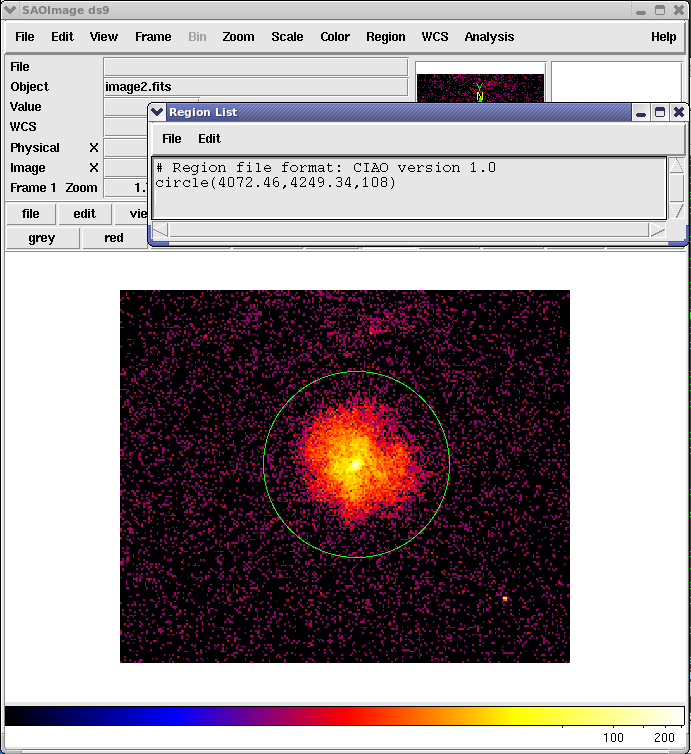### Figure 2: Filter region defined on the image

The region displayed on the image is a circle with center at (4072.46,4249.34) and a radius of 108 (using the physical coordinate system). This region will be used to fit the source.

After the desired region size is set, as shown in Figure 2, use the RegionList Regions menu to get the region definition, first setting the format to CIAO and the coordinate system to Physical in the menu that pops up:

```# Region file format: CIAO version 1.0
circle(4072.46,4249.34,108)
```

The region may also be saved to a file; see the DS9 thread for instructions.

### Define the filter on the command line

Sherpa and the DS9 session opened with image_data can interact with each other, so that the defined region may be displayed on on the Sherpa command-line with the image_getregion function.

```sherpa> image_getregion(coord="physical")
'circle(4072.46,4249.34,108);'
```

The image_getregion command be default returns region strings in logical/image coordinates, so the coordinates must be explicitly set to "physical".

The filter is now set on the command-line with the region definition:

```sherpa> notice2d("circle(4072.46,4249.34,108)")
```

or by formatting the string returned by image_getregion with the Python replace string method:

```sherpa> notice2d(image_getregion(coord="physical").replace(";",""))
```

One may also specify a FITS or ASCII file which contains the region definition:

```sherpa> notice2d("filter.reg")
```

Using the image_data() command again displays the filtered data, as shown in Figure 3.

### Figure 3: Filtered data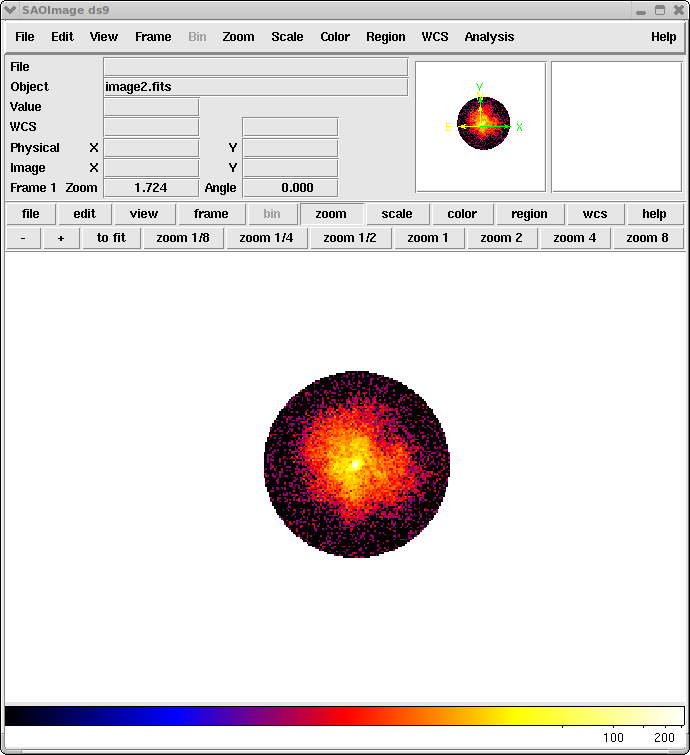### Figure 3: Filtered data

This image shows the data that is to be fit (again using a logarithmic scaling to display the full dynamic range of the data). The excluded pixels have been set to NaN which DS9 displays as the background color of white (using its default preference settings).

### Load filter information from file

As of the release of Sherpa version 2 in CIAO 4.2, it is possible to filter 2-D data sets with filter information read from FITS image files using the load_filter function. The filter image input to load_filter should contain 1s and 0s to indicate which pixels should be noticed/ignored, and should match the source image in shape and number of pixels. When the new ignore argument of load_filter is set to False (default), the pixels in the data image which correspond to those marked by 1s in the filter image will be noticed, and the reverse when ignore is True.

The filter information contained in a FITS image filter file would be applied to the source image data set using the load_filter function as follows:

```sherpa> load_filter("load_filter_image.fits", ignore=False)
```

An example of a FITS image file which can be used to filter the source image might look like that shown in Figure 4, where the pixels to be noticed are those which have a value of 1 (because ignore=False), and those which should be excluded from the analysis are the pixels with a value of 0.

### Figure 4: FITS image file containing filter information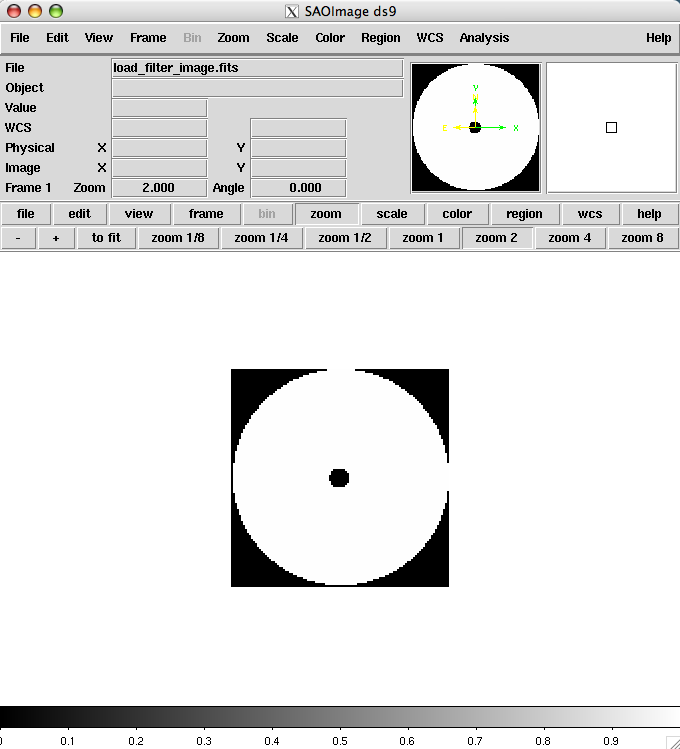### Figure 4: FITS image file containing filter information

The filter contained in the example FITS image filter file load_filter_image.fits excludes from the analysis the pixels in the central point source region, and those in the background region surrounding the apparent extent of the diffuse source emission.

## Defining a Source Model

We wish to fit the image using a source model expression that involves multiple model components. By the end of the thread, we will use two two-dimensional Gaussian functions together with a constant background component, but for now we begin with only one Gaussian.

Since this is an example, these choices are not physically motivated but just an empirical choice to describe the emission.

```sherpa> set_source(gauss2d.g1)
```

It may be necessary to modify the initial parameter values and boundaries before performing the fit. For complex models, physical knowledge of the problem can guide the initial settings of parameters and their ranges, and improve the final convergence of the fit. For simple, single-component models, the Sherpa guess function may be used to automatically set sensible starting values and ranges for the model parameters. To have Sherpa automatically query for the initial parameter values when a model is established, set 'paramprompt(True)' (the default value is False).

Note that the ellipticity and its angle (theta) are already frozen, so they do not vary during the fit.

```sherpa> g1.ampl = 20
sherpa> g1.fwhm = 20
sherpa> g1.xpos = 4065.5
sherpa> g1.ypos = 4250.5
```

The upper-limits of the fwhm, xpos, and ypos parameters are constrained to roughly the size of the image in physical coordinates (300). ampl is restricted to the range 1:1000.

```sherpa> g1.fwhm.max = 4300
sherpa> g1.xpos.max = 4300
sherpa> g1.ypos.max = 4300
sherpa> g1.ampl.min = 1
sherpa> g1.ampl.max = 1000
```

The current source model definition may be displayed:

```sherpa> show_model()
Model: 1
gauss2d.g1
Param        Type          Value          Min          Max      Units
-----        ----          -----          ---          ---      -----
g1.fwhm      thawed           20  1.17549e-38         4300
g1.xpos      thawed       4065.5 -3.40282e+38         4300
g1.ypos      thawed       4250.5 -3.40282e+38         4300
g1.ellip     frozen            0            0        0.999
g1.theta     frozen            0     -6.28319      6.28319    radians
g1.ampl      thawed           20            1         1000
```

The image_fit command displays a montage of the data, current model, and residuals that can be used to see how well the model describes the data.

We decide to include a background component before fitting, and so add in a constant model to the source expression. The value of the background model (0.2) was estimated from a radial profile. The upper limit is set to 100 as a reasonable constraint.

```sherpa> set_source(g1+const2d.bgnd)
sherpa> bgnd.c0 = 0.2
sherpa> bgnd.c0.max = 100
```

The model is now:

```sherpa> show_model();
Model: 1
(gauss2d.g1 + const2d.bgnd)
Param        Type          Value          Min          Max      Units
-----        ----          -----          ---          ---      -----
g1.fwhm      thawed           20  1.17549e-38         4300
g1.xpos      thawed       4065.5 -3.40282e+38         4300
g1.ypos      thawed       4250.5 -3.40282e+38         4300
g1.ellip     frozen            0            0        0.999
g1.theta     frozen            0     -6.28319      6.28319    radians
g1.ampl      thawed           20            1         1000
bgnd.c0      thawed          0.2 -3.40282e+38          100
```

## Fitting the model

We are now ready to fit the data:

```sherpa> fit()
Dataset               = 1
Statistic             = cash
Initial fit statistic = 20661.5
Final fit statistic   = -48907.8 at function evaluation 529
Data points           = 9171
Degrees of freedom    = 9166
Change in statistic   = 69569.3
g1.fwhm        57.9477
g1.xpos        4070.4
g1.ypos        4251.11
g1.ampl        23.3562
bgnd.c0        0.266365
```

The best-fit values for the Gaussian's FWHM and amplitude, and the background level are close to the values we guessed previously. Since we are using the PHYSICAL coordinate system the xpos, ypos, and fwhm parameters are given in the physical system. For this ACIS image this is the SKY coordinate system, which means the FWHM corresponds to 57.8 * 0.492 = 28.5 arcseconds. This can be calculated at the Sherpa prompt since we can easily access parameter values:

```sherpa> g1.fwhm.val * 0.492
```Using model parameter values

If g1.fwhm * 0.492 is just used, then the desired answer is not returned; the .val suffix for the parameter name is required to return the value at the Sherpa prompt.

The fit can be visualised using image_fit, which displays the data, model, and residual image. Here we are interested in just the residuals:

```sherpa> image_resid()
```

### Figure 5: Residuals of the best-fit model using: g1+bgnd### Figure 5: Residuals of the best-fit model using: g1+bgnd

The residual image of the best-fitting two-dimensional Gaussian plus constant model. The source is obviously more complex than this description: an excess in the core is visible as well as spatially-correlated residuals (e.g. regions of positive or negative pixels) at larger scales.

The horizontal and vertical graphs—accessed from the DS9 View menu—plot the data along the crosshair axes. The color scale has also been changed from that used in Figure 3.

The resulting image is shown in Figure 5. Although the Gaussian does a reasonable job at describing the radial profile of the large-scale emission, the residuals do appear to be spatially correlated. This suggests that the model used here is insufficient to describe all the structure in the source; however, it will suffice for this example.

The save_model and save_resid functions may be used to save the 2-D fit results to FITS image files:

```sherpa> save_model("model.fits")

sherpa> save_resid("resid.fits")
```

Here, save_model saves the model array associated with 2-D data set id=1 to the FITS image file model.fits, and the save_resid function saves the simple data-minus-model counts residuals array to the FITS image file resid.fits.

### Calculating errors on the fit parameters

Note that the statistic value is negative because we are using the Cash statistic—which is a maximum-likelihood statistic—rather than a χ2 statistic. This means that we cannot get an "absolute" measure of the fit—i.e. how well the model actually fits the data. However, we can still use the conf command to estimate errors on these parameters, since this relies on the change in the statistic value rather than the absolute value:

```sherpa> conf()
g1.ypos lower bound:	-0.178832
g1.fwhm lower bound:	-0.28347
g1.ypos upper bound:	0.178832
g1.fwhm upper bound:	0.28347
g1.xpos lower bound:	-0.1807
g1.xpos upper bound:	0.1807
g1.ampl lower bound:	-0.243301
g1.ampl upper bound:	0.245803
bgnd.c0 lower bound:	-0.00931359
bgnd.c0 upper bound:	0.00946505
Dataset               = 1
Confidence Method     = confidence
Iterative Fit Method  = None
Statistic             = cash
confidence 1-sigma (68.2689%) bounds:
Param            Best-Fit  Lower Bound  Upper Bound
-----            --------  -----------  -----------
g1.fwhm           57.9477     -0.28347      0.28347
g1.xpos            4070.4      -0.1807       0.1807
g1.ypos           4251.11    -0.178832     0.178832
g1.ampl           23.3562    -0.243301     0.245803
bgnd.c0          0.266365  -0.00931359   0.00946505
```

The residual image and profile of the fit show excess emission at the center of the source. We will add another Gaussian component to try and account for this emission. The upper parameter limits used for g1 are also used for g2.

```sherpa> freeze(g1)

sherpa> set_source(gauss2d.g2+g1+bgnd)
sherpa> g2.fwhm = 10
sherpa> g2.ampl = 100
sherpa> g2.xpos = 4065.5
sherpa> g2.ypos = 4250.5
sherpa> g2.fwhm.max=4300
sherpa> g2.xpos.max=4300
sherpa> g2.ypos.max=4300
sherpa> g2.ampl.min=1
sherpa> g2.ampl.max=1000

sherpa> fit()
Dataset               = 1
Statistic             = cash
Initial fit statistic = -50788.3
Final fit statistic   = -53918.2 at function evaluation 562
Data points           = 9171
Degrees of freedom    = 9166
Change in statistic   = 3129.92
g2.fwhm        6.08634
g2.xpos        4070.78
g2.ypos        4249.34
g2.ampl        228.721
bgnd.c0        0.260454
```

Since the g1 model was frozen, those values did not change in the fit:

```sherpa> show_model()
Model: 1
((gauss2d.g2 + gauss2d.g1) + const2d.bgnd)
Param        Type          Value          Min          Max      Units
-----        ----          -----          ---          ---      -----
g2.fwhm      thawed      6.08634  1.17549e-38         4300
g2.xpos      thawed      4070.78 -3.40282e+38         4300
g2.ypos      thawed      4249.34 -3.40282e+38         4300
g2.ellip     frozen            0            0        0.999
g2.theta     frozen            0     -6.28319      6.28319    radians
g2.ampl      thawed      228.721            1         1000
g1.fwhm      frozen      57.9477  1.17549e-38         4300
g1.xpos      frozen       4070.4 -3.40282e+38         4300
g1.ypos      frozen      4251.11 -3.40282e+38         4300
g1.ellip     frozen            0            0        0.999
g1.theta     frozen            0     -6.28319      6.28319    radians
g1.ampl      frozen      23.3562            1         1000
bgnd.c0      thawed     0.260454 -3.40282e+38          100
```

To see if the central component is elliptical—as suggested by the earlier residual image (Figure 5)—we "thaw" the ellipticity and position-angle parameters of the second Gaussian. They are set to non-zero values to help the fit.

```sherpa> thaw(g2.ellip)
sherpa> thaw(g2.theta)
sherpa> g2.ellip = 0.1
sherpa> g2.theta = 1

sherpa> fit()
Dataset               = 1
Statistic             = cash
Initial fit statistic = -53955.8
Final fit statistic   = -54058.2 at function evaluation 570
Data points           = 9171
Degrees of freedom    = 9164
Change in statistic   = 102.481
g2.fwhm        7.42264
g2.xpos        4070.73
g2.ypos        4249.34
g2.ellip       0.315905
g2.theta       0.803424
g2.ampl        230.245
bgnd.c0        0.260308
```

The residuals of this fit are shown in Figure 6.

### Figure 6: Residuals of the best-fit model using: g1+g2+bgnd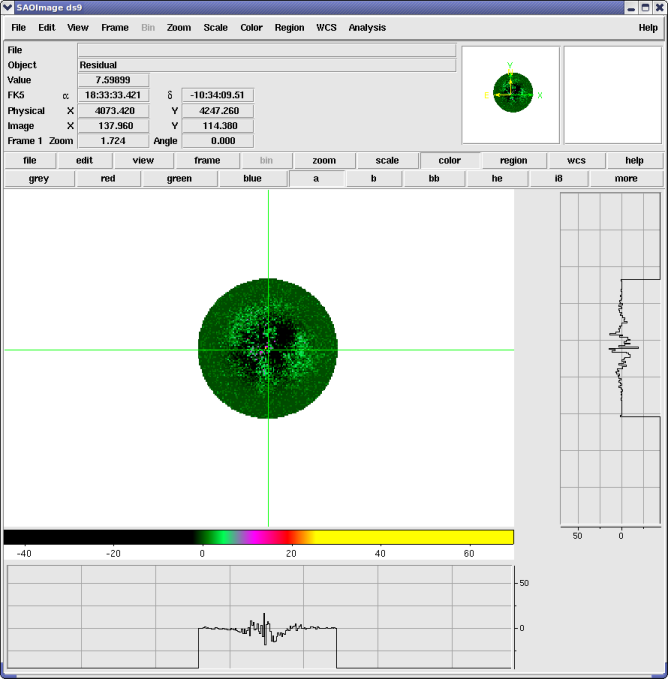### Figure 6: Residuals of the best-fit model using: g1+g2+bgnd

Using two Gaussians together with a constant provides a better model of the data than Figure 5. However, we note that the residual image still shows correlated areas. You could add further model components to the fit to account for these regions, or decide to use a different set of functions to try and describe the emission.

To see how reliable the ellipticity and theta values are, we calculate the 1σ errors using the conf() method. In this case we explicitly list the parameters we are interested in (g2.ellip and g2.theta) to avoid calculating the limits for the other parameters.

```sherpa> conf(g2.ellip, g2.theta)
g2.theta lower bound:	-0.0399632
g2.ellip lower bound:	-0.0222023
g2.ellip upper bound:	0.0215189
g2.theta upper bound:	0.0402757
Dataset               = 1
Confidence Method     = confidence
Iterative Fit Method  = None
Statistic             = cash
confidence 1-sigma (68.2689%) bounds:
Param            Best-Fit  Lower Bound  Upper Bound
-----            --------  -----------  -----------
g2.ellip         0.315905   -0.0222023    0.0215189
g2.theta         0.803424   -0.0399632    0.0402757
```

We can use the image_source_component command to image one, or a combination of, individual unconvolved source model components in order to visualize the contribution to the full model being used to fit the image data.Tip

The image_model_component command is available for visualizing PSF-convolved model components, and get_model_component_image/get_source_component_image for accessing the data arrays and preferences which define the corresponding image.

Here we image the extra g2 Gaussian component to quickly visualize its contribution to the fit of the emission.

```sherpa> image_source_component(g2)
```

The g2 model component image is shown in Figure 7.

### Figure 7: The g2 model contribution to the best-fit model g1+g2+bgnd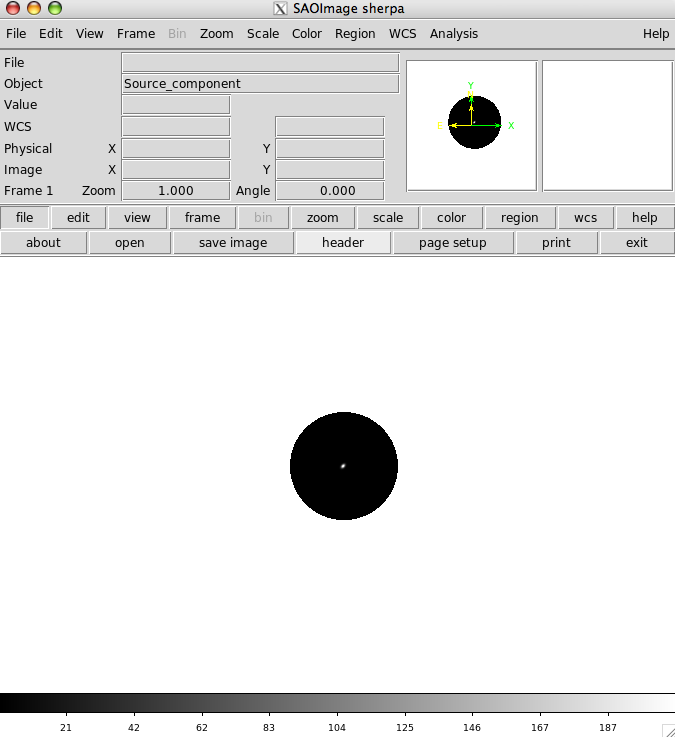### Figure 7: The g2 model contribution to the best-fit model g1+g2+bgnd

An image of the g2 model contribution to the fit of the emission using best-fit model g1+g2+bgnd.

## Checking the Sherpa session

The final overall status of this Sherpa session may be viewed as follows:

```sherpa> show_all()
Data Set: 1
Filter: Circle(4072.46,4249.34,108)
name      = image2.fits
x0        = Float64
x1        = Float64
y         = Float64
shape     = (216, 261)
staterror = None
syserror  = None
sky       = physical
crval    = [ 3798.5, 4019.5]
crpix    = [ 0.5, 0.5]
cdelt    = [ 2., 2.]
eqpos     = world
crval    = [ 278.386 , -10.5899]
crpix    = [ 4096.5, 4096.5]
cdelt    = [-0.0001, 0.0001]
crota    = 0
epoch    = 2000
equinox  = 2000
coord     = physical

Model: 1
((gauss2d.g2 + gauss2d.g1) + const2d.bgnd)
Param        Type          Value          Min          Max      Units
-----        ----          -----          ---          ---      -----
g2.fwhm      thawed      7.42264  1.17549e-38         4300
g2.xpos      thawed      4070.73 -3.40282e+38         4300
g2.ypos      thawed      4249.34 -3.40282e+38         4300
g2.ellip     thawed     0.315905            0        0.999
g2.theta     thawed     0.803424     -6.28319      6.28319    radians
g2.ampl      thawed      230.245            1         1000
g1.fwhm      frozen      57.9477  1.17549e-38         4300
g1.xpos      frozen       4070.4 -3.40282e+38         4300
g1.ypos      frozen      4251.11 -3.40282e+38         4300
g1.ellip     frozen            0            0        0.999
g1.theta     frozen            0     -6.28319      6.28319    radians
g1.ampl      frozen      23.3562            1         1000
bgnd.c0      thawed     0.260308 -3.40282e+38          100

name         = simplex
ftol         = 1.19209289551e-07
maxfev       = None
initsimplex  = 0
finalsimplex = 9
step         = None
verbose      = 0

Statistic: Cash
Maximum likelihood function.

Counts are sampled from the Poisson distribution, and so the best
way to assess the quality of model fits is to use the product of
individual Poisson probabilities computed in each bin i, or the
likelihood L:

L = (product)_i [ M(i)^D(i)/D(i)! ] * exp[-M(i)]

where M(i) = S(i) + B(i) is the sum of source and background model
amplitudes, and D(i) is the number of observed counts, in bin i.

The Cash statistic _ is derived by (1) taking the logarithm of
the likelihood function, (2) changing its sign, (3) dropping the
factorial term (which remains constant during fits to the same
dataset), and (4) multiplying by two:

C = 2 * (sum)_i [ M(i) - D(i) log M(i) ]

The factor of two exists so that the change in cash statistic from
one model fit to the next, (Delta)C, is distributed approximately
as (Delta)chi-square when the number of counts in each bin is
high.  One can then in principle use (Delta)C instead of
(Delta)chi-square in certain model comparison tests. However,
unlike chi-square, the cash statistic may be used regardless of
the number of counts in each bin.

The magnitude of the Cash statistic depends upon the number of
bins included in the fit and the values of the data
themselves.  Hence one cannot analytically assign a
goodness-of-fit measure to a given value of the Cash statistic.
Such a measure can, in principle, be computed by performing
Monte Carlo simulations. One would repeatedly sample new
datasets from the best-fit model, fit them, and note where the
observed Cash statistic lies within the derived distribution
of Cash statistics. Alternatively, the `cstat` statistic can
be used.

Notes
-----
The background should not be subtracted from the data when this
statistic is used.  It should be modeled simultaneously with the
source.

The Cash statistic function evaluates the logarithm of each data
point. If the number of counts is zero or negative, it's not
possible to take the log of that number. The behavior in this case
is controlled by the `truncate` and `trunc_value` settings in the
.sherpa.rc file:

- if `truncate` is `True` (the default value), then
`log(trunc_value)` is used whenever the data value is <= 0.  The
default is `trunc_value=1.0e-25`.

- when `truncate` is `False` an error is raised.

References
----------

..  "Parameter estimation in astronomy through application of
the likelihood ratio", Cash, W. 1979, ApJ 228, 939

Fit:Dataset               = 1
Statistic             = cash
Initial fit statistic = -53955.8
Final fit statistic   = -54058.2 at function evaluation 570
Data points           = 9171
Degrees of freedom    = 9164
Change in statistic   = 102.481
g2.fwhm        7.42264
g2.xpos        4070.73
g2.ypos        4249.34
g2.ellip       0.315905
g2.theta       0.803424
g2.ampl        230.245
bgnd.c0        0.260308

Confidence:Dataset               = 1
Confidence Method     = confidence
Iterative Fit Method  = None
Statistic             = cash
confidence 1-sigma (68.2689%) bounds:
Param            Best-Fit  Lower Bound  Upper Bound
-----            --------  -----------  -----------
g2.ellip         0.315905   -0.0222023    0.0215189
g2.theta         0.803424   -0.0399632    0.0402757
```

## Scripting It

The commands used to run this thread may be saved in a text file such as fit.py, which can then be executed as a script with exec(open("fit.py").read()). Alternatively, the Sherpa session can be saved to a binary file with the save command (restored with the restore command), or to an editable ASCII file with save_all.

The Sherpa script command may be used to save everything typed on the command line in a Sherpa session:

```sherpa> script(filename="sherpa.log", clobber=False)
```

(Note that restoring a Sherpa session from such a file could be problematic since it may include syntax errors, unwanted fitting trials, et cetera.)

The CXC is committed to helping Sherpa users transition to new syntax as smoothly as possible. If you have existing Sherpa 3 scripts or save files, submit them to us via the CXC Helpdesk and we will provide the CIAO/Sherpa 4.11 syntax to you.

## Summary

In this thread, we have shown you how you can fit a two-dimensional model to your image data. As with fitting one-dimensional data, care must be taken to avoid reaching a local, rather than global, minimum. It is suggested that you fit your data using different optimization methods—for instance the fit above could be re-done with the Monte Carlo ("moncar") method—and to try different initial parameter values when fitting.

In the example above, the fit was not very complex: possible additions would have been to link the centers of the two Gaussians to be the same; simultaneously fit the same model to more than one dataset (e.g. for multiple observations of a source or when analysing the data from the three imaging cameras in XMM-Newton); include an exposure map in the fit to account for instrumental features such as chip gaps and bad columns; or convolve the model by the PSF during fitting.

## History

 15 May 2008 rewritten for Sherpa Beta 16 Jul 2008 minor update to use simplex rather than the neldermead optimisation method as they are the same 11 Dec 2008 updated for CIAO 4.1 05 Jan 2010 updated for CIAO 4.2 07 Jun 2010 updated to include save_model and save_resid functions for saving 2-D fit results 12 Jun 2010 updated for CIAO 4.2 Sherpa v2: load_filter now accepts filter files in FITS image format for filtering 2-D data sets. S-Lang version of thread removed 15 Dec 2010 updated for CIAO 4.3: the new image_source_component function is available for visualizing the contribution of individual unconvolved model components to a fit 30 Jan 2012 reviewed for CIAO 4.4 (no changes) 05 Dec 2013 reviewed for CIAO 4.6: no changes 09 Dec 2014 reviewed for CIAO 4.7: updated example file and fit results 31 Oct 2016 reviewed for CIAO 4.9: updated fit results 12 Dec 2018 reviewed for CIAO 4.11, no content change